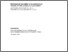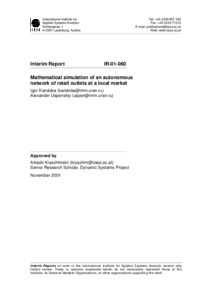# Mathematical Simulation of an Autonomous Network of Retail Outlets at a Local Market

Kandoba, I. & Uspenskiy, A. (2001). Mathematical Simulation of an Autonomous Network of Retail Outlets at a Local Market. IIASA Interim Report. IIASA, Laxenburg, Austria: IR-01-060Preview Text IR-01-060.pdf Download (161kB) | Preview

## Abstract

The paper is devoted to economic and mathematical simulation of an autonomous network of retail outlets at a local market. The question of simulation of redistribution precesses in the network is discussed. Two interconnected and complemented economic -mathematical models are suggested. These models describe the evolution precesses of the sale conditions in the network. They are constructed on the basis of the price demand and the system state functions. The mathematical models are described by a dynamic system of partial differential equations. The main attention is given to mathematical simulation of the redistribution process of consumer demand through retail outlets in the network under fluctuations of prices. The numerical realization of the models is discussed. Computer simulations show that the proposed models can adequately reflect some real processes of the sales redistribution in the network.

Item Type: Monograph (IIASA Interim Report) Dynamic Systems (DYN) IIASA Import 15 Jan 2016 02:13 27 Aug 2021 17:17 https://pure.iiasa.ac.at/6464View Item